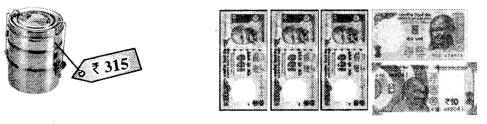# PSEB 4th Class Maths Solutions Chapter 4 Money (Currency) Ex 4.2

Punjab State Board PSEB 4th Class Maths Book Solutions Chapter 4 Money (Currency) Ex 4.2 Textbook Exercise Questions and Answers.

## PSEB Solutions for Class 4 Maths Chapter 4 Money (Currency) Ex 4.2

1. Add the amount of the following currency notes:

Question 1.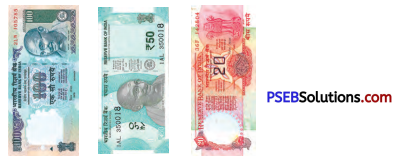Solution:
₹ 170

Question 2.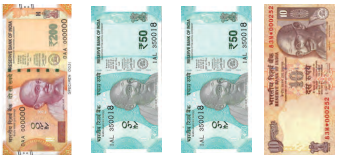Solution:
₹ 310Question 3.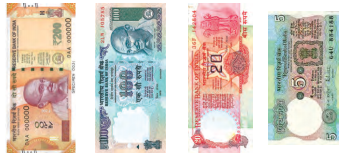Solution:
₹ 525

Question 4.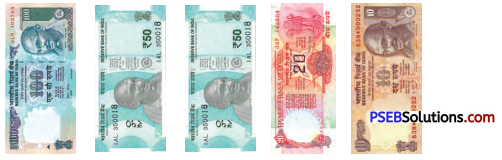Solution:
₹ 230

Question 5.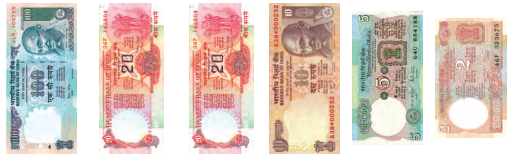Solution:
₹ 157

2. Find the sum of following amounts :

Question 1.
₹ 200, ₹ 50, ₹ 20
Solution:
₹ 200, ₹ 50, ₹ 20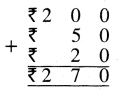Question 2.
₹ 350, ₹ 165, ₹ 75
Solution:
₹ 350, ₹ 165, ₹ 75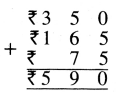Question 3.
₹ 470, ₹ 105, ₹ 55
Solution:
₹ 470, ₹ 105, ₹ 55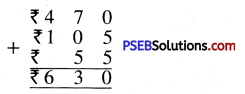Question 4.
₹ 250, ₹ 90, ₹ 110
Solution:
₹ 250, ₹ 90, ₹ 110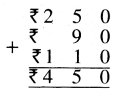Question 5.
₹ 200, ₹ 160, ₹ 50
Solution:
₹ 200, ₹ 160, ₹ 50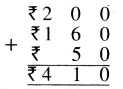3. Find the difference (subtract) of following amounts :

Question 1.
₹ 200 and ₹ 150
Solution:
₹ 200. ₹ 150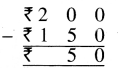Question 2.
₹ 450 and ₹ 200
Solution:
₹ 450, ₹ 200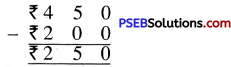Question 3.
₹ 500 and ₹ 270
Solution:
₹ 500, ₹ 270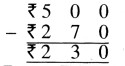Question 4.
₹ 120 and ₹ 75
Solution:
₹ 120, ₹ 75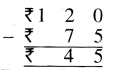Question 5.
₹ 300 and ₹ 125
Solution:
₹ 300, ₹ 125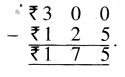4. Put (✓) on the currency note or coins which are required for buying given things :

Question 1.Solution: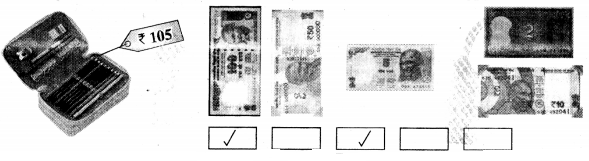Question 2.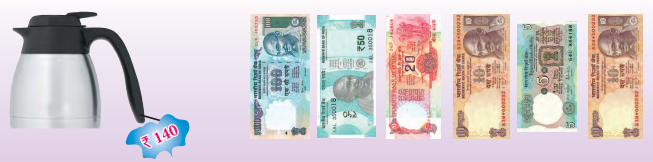Solution: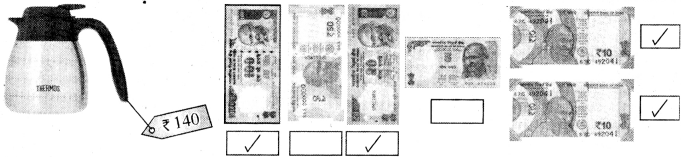Question 3.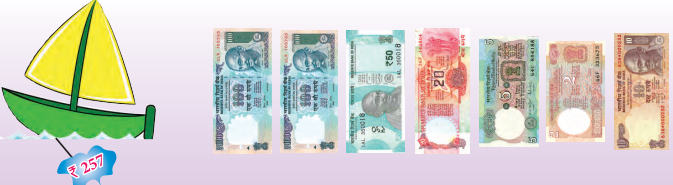Solution: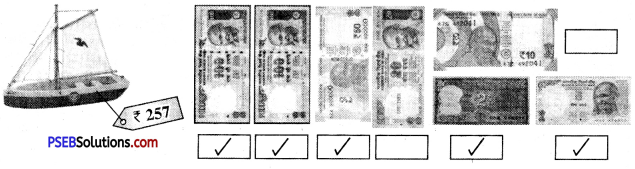Question 4.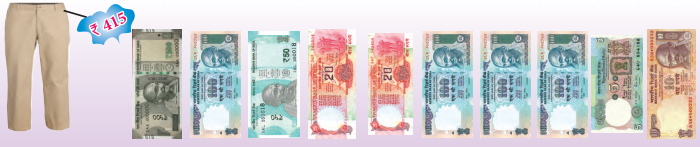Solution: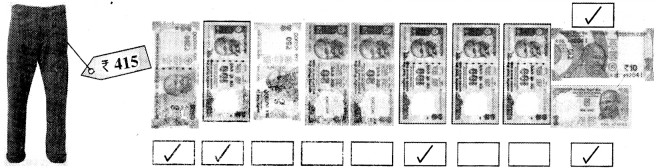Question 5.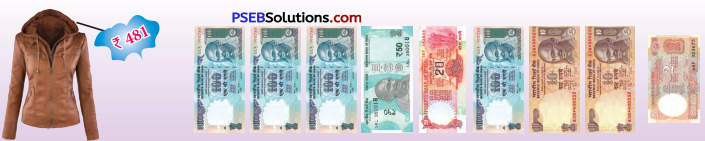Solution: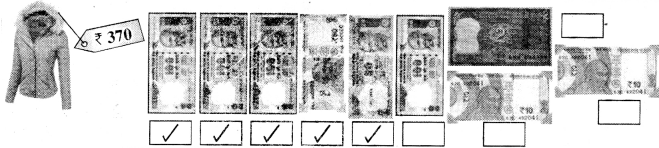5. Draw required currency notes and coins for buying things as tender.

Question 1.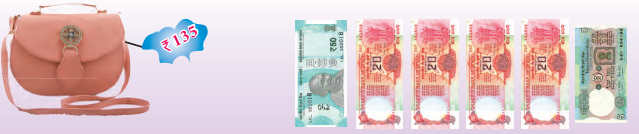Solution: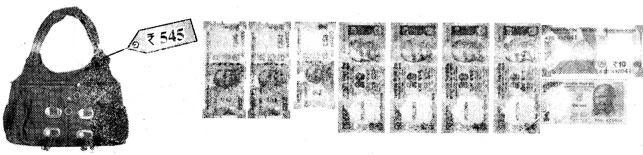Question 2.Solution: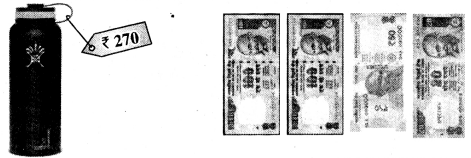Question 3.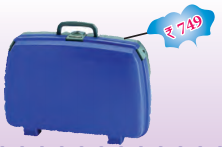Solution: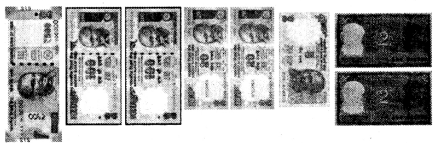Question 4.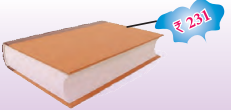Solution: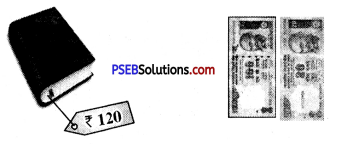Question 5.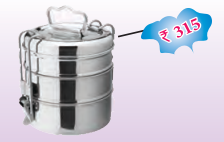Solution: